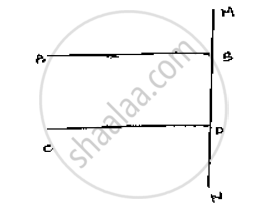Share
Notifications

View all notifications
Books Shortlist
Your shortlist is empty

# Prove that the Straight Lines Perpendicular to the Same Straight Line Are Parallel to One Another. - CBSE Class 9 - Mathematics

Login
Create free account

Forgot password?
ConceptParallel Lines and a Transversal

#### Question

Prove that the straight lines perpendicular to the same straight line are parallel to one
another.

#### Solution

Let  AB and CD perpendicular to the Line MN∠ABD = 90°            [∵ AB  ⊥ MN ]     ......(i )

∠CON = 90°          [ ∵ CD ⊥ MN]      ......(ii)

Now,

∠ABD = ∠CDN = 90°                    [From (i) and (ii)]

AB || CD, Since corresponding angles are equal.

Is there an error in this question or solution?

#### APPEARS IN

Solution Prove that the Straight Lines Perpendicular to the Same Straight Line Are Parallel to One Another. Concept: Parallel Lines and a Transversal.
S